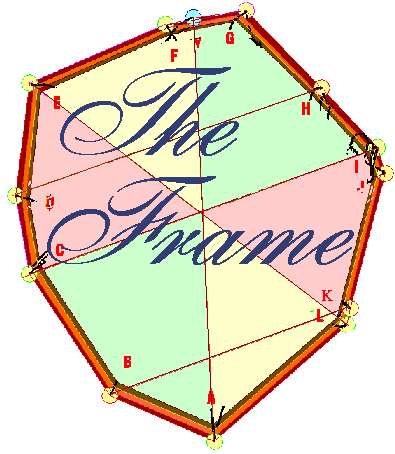Top The Nazca Monkey

Connect the Dots

Drawing the external boundary for the Stone Age Athena-engraving is rather easy due to the existence of clear main peripheral points. These thirteen points are then connected  by lines. This is the 'Frame' - as simple a step as can be towards checking the image for hints of planned layout.

Beginner's luck

It was 1985, at the end of Stone-Age for computing masses. I was one of the primitives with no scanner, nor computer to help me with graphics. Life-size copies of the picture were too small for me to do accurate work on with the classic ruler and compasses, and so I got a sheaf of 2:1 blow-ups from the nearby printers. Did the ancient agency foresee that someone will resort to just that one day, due to limitations of human vision?  One way or another, it's reasonable that the units should be Earth-commensurate.

At double-size, the engraving's units of length equal the metric system.

Now, my readings were identical to the designer's intended values!

The measurements were rounded to the nearest millimeter, the finest detail available on my ruler.The Game of Quotes

At the first glance, the thirteen whole numbers ranging from 16 to 175 - the distances between neighboring points of the Frame in millimeters - are no big deal. But then one starts learning that these numbers are simply ideal for the purpose of quoting Pi, Phi, and rates of Equinoctial Precession as many times as possible, and as far as the following:

Pi   =  3.1415926535897932384626433832..     twenty-eight decimals
Phi =  1.6180339887..                                        ten decimals
Equinoctial Precession  -  rates match today's state of the art measurements.

I'm convinced that no other set of thirteen whole numbers in a comparable range can rival the Frame at this type of communication. Clearly, its designers had to be highly sophisticated, and also in possession of astronomical instruments at least equal to what we have now.
Could the Frame just occur by chance? In that case - how many possible combinations are there of thirteen whole numbers in the range from about 10 to about 180, as long as their total falls somewhere between 1,000 and 1,300, and numbers can appear more than once?
Given such conditions, any mathematician realizes that the odds against coming across the Frame by accident are mind-boggling. There are sextillions of such groupings of numbers.
But the order in which segments appear in those groupings matters as well. Each of the sextillions of sets of thirteen segments can be arranged in
6,227,020,800 ways.
If the Frame's functions cannot be improved by rearranging and replacing some, or even all of its thirteen numbers, well, then the Frame must be the best solution in its category among Nonillions (10 to the 30th power) of competing combinations possible.
This truth no naysayer dares to challenge, facing the disconcerting reality of being dumber than alleged 'blind chance'.

The Game Rules and Pieces

We can scramble our Frame into some 4,000 unique combinations of segments, but we can break it up into only 156 unique pieces between two Frame points (a piece is either
a single segment, or a sequence of neighbouring segments)That's where we ought to look for rational meaning.
In addition, there is something called 'the Strong Connection' between Frame points B and G. This connection proves of great importance in a number of ways. Consequently, it counts in with additional Frame sequences. This, I believe, adds another 96 combinations. If so, there are 252 total combinations
Such sequences of segments make sense when subjected to simple manipulation like addition, subtraction, multiplication, or division.

The rule is that any
gamepiece for the next move must be either a part of the present  gamepiece, or immediately adjacent to it. Segments connected across the Frame by the points B and G (16 & 175 across to 113 & 146)  are considered immediately adjacent, as well ( as if through subspace, a worm-hole ).

We can look at the Frame's numbers in at least four ways:

1) as is
2) ordered by segment size
3) ordered by unique segment size
4) as the segments appear on
the 'Wheel of 113' (a pie-chart of 113's moduli)

Opening Moves (beginner level)

The
Frame serves as the doorway into the picture, but there must be something to entice us into entering, some attention-getter, a sign above the door. Sure enough, no one can miss

the Section of Regular Proportions

 27 54 108 81 27

The section of five segments spanning the points H to M exhibits regular proportions!
These proportions,  1-2-4-3-1, as well as the actual numbers - all multiples of 9 - look deliberate.

The Frame lends itself to transformation into a pie-chart:

81 & 27

The four segments to the left of 27 add up to 270, and the eight segments to the right of 81 add up to 810 -  two segments and their tenfold multiples side by side.
The twelve segments involved add up to 1080, a tenfold multiple of 108, which we have twice (108 & 81 + 27), twenty-fold of 54, and forty-fold of 27 - all these distances are inside the stretch of 1080.Below: And then we see more regular proportions: two neighboring sections measure 340 each - those are followed by two sections of 108 each. A middle segment in the section of three (80-113-146) equals one third of that section, etc. Who'd guess that looking into ratios among these segments will be so entertaining?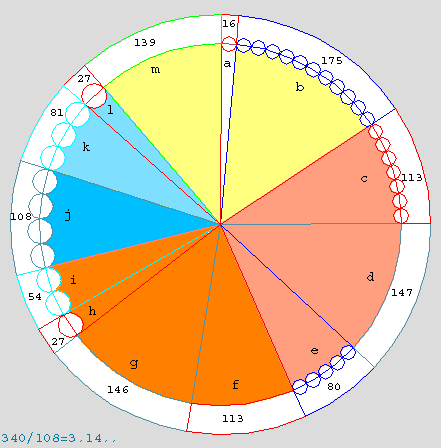Pi & Phi

The number 16 could represent the first two digits of Phi, the acclaimed Golden Ratio, and it is the base of the hexadecimal system.
A check of the next door neighbors of 16, reveals that they total 314. Of course, those are the first three digits of Pi, the best known ratio of all.
Phi is embraced by Pi here. Is this a sign of things to come?

 139 16 175

Without these three segments, the entire rest of the Frame forms several Pi proportions. In efffect, the above quote of Pi represents an answer to the question posed by those proportions!

T
wice 340 (680), next to twice 108 (216) The arrangement is shown below.

 113 147 80
 113 146 27 54

 108
 81 27

What is the ratio between the groups?

Pi (3.14.....)

680/216 =  3.14...  The first three digits are those of Pi.

Pi (3.14.....)

340/108 =  3.14...

That's two approximations of Pi (680/216, and 340/108) in one fell swoop.

The second 340-section (clockwise) is next to the segment of 80. Their total of 420 also has a factor pair of 3 x 140, or 314 without the multiplication symbol.the Question & the Answer

The open ended Pi sequence of ten consecutive segments adjoins the segment of 139 on one end, and the segment of 175 on the other. Those two segments total 314, the first three digits of Pi.

360 degrees of order

After the above mentioned 314 comes 16, the last segment left in the circuit.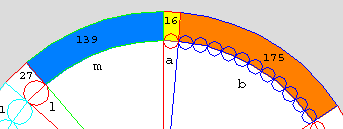314 & 16, or 31416 - Pi rounded to five digits. The entiree Frame circuit is devoted to Pi!

Quoting Pi to twenty-eight decimals

Just by themselves, the last three segments (139 & 16 & 175) enable a somewhat refined result.

175 +  139       = 314

175  -   16       =  159      314 159 the first six digits of Pi

The arangement is planned, and there is a lot more confirmation still to come.
The immediate vicinity of 16 & 175 is rife with Pi relations.

First of all, there is yet another way here to arrive to the above result, and beyond. We worked with 16 & its short wings, now we begin from:

16 & two segments to its left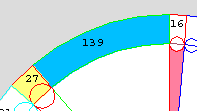27 & 139 millimeters translated to centimeters in whole numbers becomes 3 & 14

314
__ the first three digits of Pi

Of course, 16 is next: 3-14-16..   Pi rounded to four decimals, but we are after a greater prize.

16 & 175 & two segments on each side

 27 139 16 175 113 147

3                 14                     159   (175-16)                    260  (113+147)

After 27 & 139 (3 & 14), there come the Strong Connection segments 16 & 175. The difference between the latter is 159:

3 14 159          the first six digits of Pi

After 16 & 175, we have the pair of 113 & 147, which totals 260 millimeters, or 26 centimeters.

3 14 159 26
the first eight digits of Pi

Pi (3.1415926..)

Composite Numbers and  Phi

Before going on with Pi, let's take a look how the very same location also delves into Phi.
Subtracting from 175 on the left helped us with the Pi's first six digits. Now, subtracting from 175 on the right (175 - 113) leaves 62 - the fraction of Phi rounded to two decimals.
Does Phi find additional support here?  The two segments to the left of 16 made a nice play on Pi. Could the two segments to the right of 16 possibly make a nice play on Phi

 16 175 113

175 + 113  = 288  = 16 x 18

So, 16 is contained
18 even times in the next two segments.  The Ancients seem to be trying to bring our attention to composite numbers. Although 288 breaks down into various multiples of whole numbers, the presence of 16 before 288 indicates that if we look at 288 here as a composite number, it should be in the following manner:

16 * 18   Without the multiplication sign:

1618the first four digits of Phi!

175 millimeters as a whole number in centimeters is 18.
In that case we have 16 followed by 18 ___ 1618 again!

Back to Pi - the Wings of 260Being on 260  (113+147) we have 175 on the left, and 80 on the right:

We just saw 175 work with 113 (and 16) as part of the idea of showing a composite number as a pair of factors:  288 = 16 x 18
175 works by itself too, as a pair of factors (35 x 5) in the idea 355/113=3.141592...
Of course, this pair of factors can be reversed to 5 x 35, instead.

175 = 5 x 35
(535)

Pi
=  3.1415926535..

The right wing of 260 is 80 millimeters or 8 centimeters long.

Pi    =    3.14159265358..

A second pair of factors in the progression

The three Pi digits after 8 are 979.

To the left of 80, from 113 to 16:

These nine segments average seventy-nine units each (9 x 79)!

113  + 146 + 27 + 54 + 108 + 81 + 27 +139 + 16 = 711 = 9 x 79

Pi    =    3.14159265358979..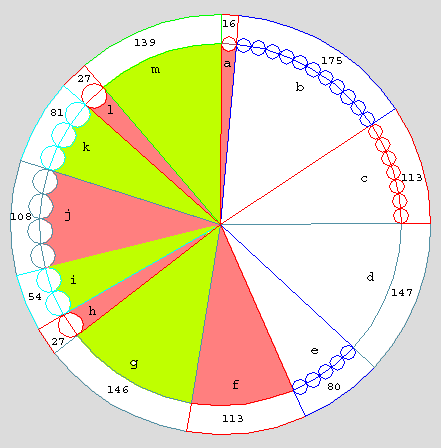In reading Pi out this far, to fifteen digits, we have covered more than the entire Frame, once again.

To extend the Pi sequence by three more digits, we need a 323. Curiously, 711 translates as either 9x79, or 3x237, and no other composite factors. That would be six digits of Pi in a row, 979 323. Unfortunately, the seventh digit, 7, is off by one; so this doesn't count.
Is there a legitimate way to get the digits 323?

Right now, we are parked on 16, at the point B. We've been here before. This is also a crossroads, where the Strong Connection cuts acrosss the Frame (image) to G, as if through subspace, a wormhole.  See more on the Strong Connection below - use this anchor.

The image below shows the B-G line connecting through the central point of the entire engraving - the origin point of the square, and apparently, of the blue pyramid's vertical axis.
This is how the Frame acquires another loop, and 16 & 175 become directly connected to  146 & 113, and likewise, to the 339-sequence of 146 & 113 & 80. The Strong Connection and the 339-sequence are going to be instrumental in all the upcoming cases of further Pi digits.It's only fitting that in the
pie-chart shown below, 16 rises symmetrically over the 339 section. The symmetry between the two is the best possible (the intervening spaces are 436 and 435).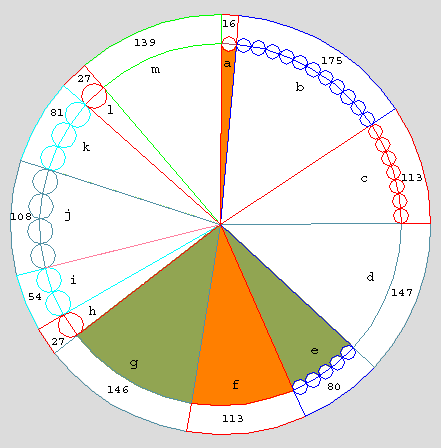So we ferry 16 over to the opposite side via the Strong Connection, in order to diminish the 339-sequence.

339 - 16  =  323        ( Later, 339+16 also plays a role.)

Pi
=    3.14159265358979323...

Next Pi digits are 8 462 64 33.. It just so happens that 80 millimeters, or 8 centimeters, part of the 339-sequence, is once more the immediate continuation

Pi    =    3.141592653589793238...

We still stand on the 339-sequence; next up are its wings.On the Wings of the 339-sequence

The three segments wedged on the right between the 339-sequence and 16, with the segment on the left of the 339 sequence, add up to 462

Pi    =    3.141592653589793238462....

And again from the 339-sequence, 80, its part, minus the 16 from across the S. Connection gives 64.

Pi
=    3.141592653589793238462
64....

Now from 16, add its two wings of 175 & 139 to it:  314 + 16 = 33(0)
We could get the same 33 within the 339 sequence by subtracting 80 from 113.

Pi    =    3.1415926535897932384626433....

A third pair of factors

Next to 139 is 27; together, the two make 166, whose factors are, among others, 83 x 2.

Pi    =    3.1415926535897932384626433832....

Our readout of Pi reaches twenty-eight Pi decimals. IThe Frame is also a mnemonic aid which enables me to remember twenty-eight decimals of Pi. That's a dramatic benefit from what naysayers allege are wild scribbles by cavemen!

Around the Frame in both Phi & Pi

We did more than a couple of complete circuits of the Frame dealing with Pi, and on top of that we got started with Phi as 1618.. The last Frame part we used was 113 (in 175+113=288 = 16 x 18).

113 & Phi

Does 113 have anything to do with Phi?

Phi = 1.6180 339 887..   where we find 113 in

339
= 113 x 3     and

887
= 1000 - 113

Judging by the above, the answer is a conditional yes. Fascinating, two Frame parts are 113s, and the Frame less those equals 1,000 units even.

Phi:

On the Strong Connection:
16 & 146 & 113

146 + 113 = 259
259 / 16 = 16.18..
1618..   the first four digits of PhiIn this position, it's hard to miss that the Strong Connection pair of 146 & 113 has wings of  27 & 54 totaling 81 on the left, and 80 on the right, whose total is :

161 ___  the first three digits of Phi

Of course, 259 divided by 161 is 1,6.. the first two digits of Phi
Our readouts here seem repetitious.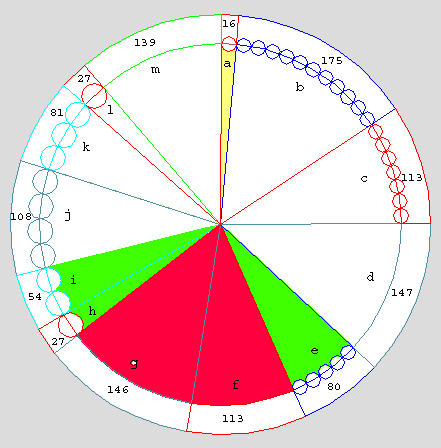Although these 81 & 80 segments are not direct neighbors, they enclose a section
which addresses the same subject as they do - Phi. Moreover, the step taken is just the first in several steps occurring in this immediate area, which produce a quote of Phi to ten decimals.. In my eyes, these facts legitimize taking this step of proceeding from a shorter quote of Phi to a more elaborate one.

So, let's write 161 down.

Of course, 80, on the right of the Strong Connection, is part of that 161.

Write down 161 & 80  ________ 161 80

80 is part of 339

Write down 161 & 80 & 339  ______
161 80 339  the first eight Phi digits

339 is surrounded by the rest of the Frame _  887   (The Frame totals 1226)

161 80 339 887   _____   the first eleven digits of Phi!
______________________________________________

Some may favor a different method of arriving at the same quote, one in which we descend through the progression:

We start with 887 & 339 ____ the Frame divided into two sections.
In the 339-section, we focus on 80, which is part of 339. That 80 with 81 to the left of 339 gives 161.  The latter method seems smoother.

887
339
80
161
Read from the bottom up   161 80 339 887    eleven digits..

More Phi

Once we get to 16180, namely 80, we note that it has a wing of 340 on its left; and as for itself, it's part of another 340 section, a direct neighbor of the first. Is 34(0) somehow significant in this context?

1618034 gives Phi rounded to seven digits.
161 80 34

There is an obvious inner divide in the image, it's the line subtending both the 339 and 887 sections.
Take a good look, from E to H, whenever this line passes near a point in the image, it then finds the point - eight consecutive times out of nine possible, and the miss is by only a little bit.This time, the already familiar 339-section  performs feats of geometry.
In the image above, the girl is shaded by a yellow umbrella - otherwise, the 339-section. The angle FGH in this section is very close to being 120 degrees. Look at the shaft of the umbrella between B and G - the Strong Connection - a perfect arrow through the bull's eyethe red crosshairs - the center of the Square . The connection divides the 120 degree angle FGH down the middle into two 60 degree angles.
Below:  Connect the center of the Square to E, the start of the 339-section. This line then creates an equilateral triangle with the lines through BG, and FG.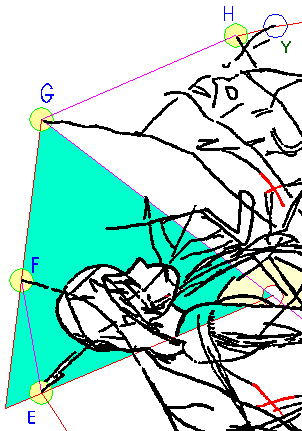How nice, the head rests on a triangle's basis, and is
about equidistant to the other two sides.
This triangle simply begs experimental completion into a hexagon centering in the 0,0 point, the center of the Square.
Thist hexagon then fits the figure from head to toe. Note the containment of the figure within the envelope shape. The center of the first equilateral triangle falls neatly onto Athena's forehead.
And later we learn that the hexagon is the offspring of two other hexagons..
see:    The HexMachineThe Strong Connection  The hexagon helps to establish the B-G connection, the Strong Connection, across the Frame: 16 & 175 on one side and 113 & 146 on the other.  Let's see some simple relationships among these values.   a)   16 & 146       16 + 146 =  162                       Phi rounded to three digits Note that 16 sports the first two digits of Phi. In that way, it's significant by itself. With the addition of 146, it's Phi rounded to three digits. That's two possible mentions of Phi, already.  b) 175 & 113       175 - 113  =   62         0.62 is Phi-minor rounded to two decimals c)  16 & 146 & 113146 + 113 = 259                     259 / 16 = 16.18..                                        1618..   the first four digits of Phi       146 & 113 & wings of 80 & 81d) In this position, it's hard to miss that 146 & 113 have 27 & 54 totalling 81  on the left and 80 on the right. The total of those is 161 ___  the first three digits of Phi  e) And 146 & 113 added up and then divided by that 161 on the wings rounds up to 1.61   _   the first three digits of Pi. f) 16 & 113 & 175 16 is connected to 175, and 113 across the Strong Connection which add up to 288 16 is contained 18 times in 288                                           1618..  the first four digits of Phi, once more e)  16 & 113 Under the circumstances, the pairing of 16 & 113 is simply sensational!                                        16  / 113 = .141592..     the Pi fraction correct to a millionth Mathematicians automatically recognize this 16 / 113 as part of an accurate, and well known, approximation of Pi. If there were a 355 number,  or 339 in addition to 16, in the neighborhood, it would make the approximation complete. The Strong Connection makes this wish come true! 80  +  113 + 146   = 3 * 113 = 339 339 is connected to 16 (339 + 16)  / 113  =   355 / 113 = 3.141592.. The diagram below makes plain that 16 is optimally balanced above the 339-section. The symmetry between the two is the best possible; count the intervening spaces (436 and 435).  It emphasizes the relationship 16 & 339, across the Strong Connection. It is then only natural to be inquisitive about the ratio between the two.In the landscape of 113s and 355s The four segments to the left of 16 add up to 355.  Here, we can read:  a) The 355-wing on the left of 16 is divided by the 113-wing of 16, across the Strong Connection:                                                                              355/113 = 3.141592... b) The section of 355 minus the 16 on the right equals the 339 across the Strong Connection. This 355 is bound with 16's wings across the strong Connection of 113, and 113 x 3 (339) .  This 355 is itself a part of a section of 452 - four multiples of 113.            16 & 139 & 27 & 81 & 108 & 54 & 27 = 452 It is then extended to seven multiples of 113 by the 339-section.                                     The ratio between 355 and 113x7 is Pi / 7                      The ratio between 355 and 113x4 is Pi / 4                      The ratio between 355 and 113x3 is Pi / 3Everything clockwise from 80 through 16 equals seven multiples of 113.  Magic squares Removing the section of seven multiples of 113, leaves 175_113_147. quote: "The magic constants of normal magic squares of order n = 3, 4, 5, … are (sequence A006003 in OEIS) 15, 34, 65, 111, 175, 260, 369, 505, 671, 870 The numbers in any row, column, or diagonal of a normal magic square form a magic series. So, 175_113_147 can be read as 175, 260 (113+147=260) just like the above series, and 147 - 113 = 34, also a value from the above series.  16 & 113 & two segments to the left The two segments to the left of both 16 and 113 combined add up to 339.                                                    146 + 27 + 27 + 139 = 339 Add the 339 to 16 and divide it by 113 to get a familiar result:                                                          3.141592   Pi to six decimals!16 & 113 & all the four values between them on the right hand sideFolding the 80 segment counter-clockwise over the next three segments  makes the distance between 16 & 113 into 355.                           435 - 80 = 355 The ratio between 355 and 113 is                                                                               3.141592..    Pi to six decimals Observation: After the last episode, 16 has compound wings of 355 both on its left and right sides, and it makes the same number 355 with 339 across the Strong Connection.       175 & 113 = 288 = Osiris number (precessional number) We already know that 113 + 175 =  16 x 18 , or 1618 - Phi in the first four digits. But, 288 is also a multiple of 36, and an Osiris Number as one-ninetieth of the Zodiac.      175 & 146  _  Maximum symmetry across the Strong Connection! We have seen maximum symmetry, for the circumstances, across the Strong Connection between 16 and  339.  That symmetry came in tandem with hidden meaning. Their Strong Connection partners _ 175 & 146 -  also have such symmetry between them, with gaps of 452mms (4 x 113) on one side, and 453mms (4 x113.25) on the other. So there should be something else to this symmetrical connection, as well, and the emphasized 113 should have something to do with it.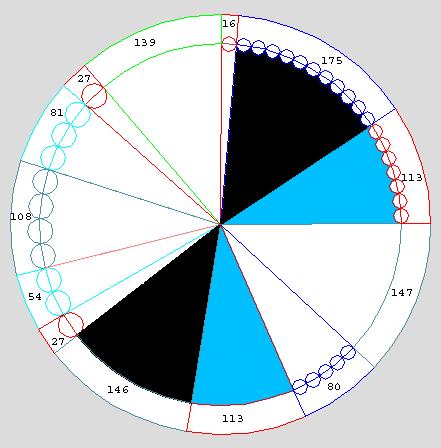175  x  146  =  25,550 On the right of both 175 and 146 we see 113. 25,550 +  226 (twice 113) equals 25,776  !!! Why would 25,776 carry an important meaning in the context of this article? At 71.6 years to precess one degree _  the full precession cycle takes 25,776 years to complete. On this first level of accuracy: the Frame Arranged by Segment Size                                                                    We can also look at the Frame as a sequence of numbers arranged by size, and then as a sequence of unique numbers arranged by size.Like this, the Frame has two clearly different sections, the numbers below 113 with the common denominator of  6,480 (one-fourth of the Zodiac), and the rest, which begins with twice 113. One is obviously meaningful, the other is not at all. Obvious:   16   27   27   54   80   81  108   All these fit the category of precessional code numbers (Osiris numbers). Unobvious:   113  113  139   146  147  175 These are divisible into two groups, each of which involves an approximation of  Pi. The two segments of 113 together :  226, multiplied by 139 approximates Pi as 31414, where the first four digits are correct.            226 * 139 = 31414        The following three segments 146, 147, 175 add up to 468, and 468 gives the decimals of Pi correct to six digits, when divided by 113                                                468/113 = 4.141592..(113 + 113) x 139 = 31414     113 + 113  + 139 = 365     _    whole days in a year     113 + 113           = 226   If we replace 31414 with the more accurate value of Pi  as 3.1416 in the equation below: 226 * 365 * 3.1416 =  25,915.0584 * 10 - approximately ten precessional cycles   365 / 226 =  1.61...  the first three digits of Phi Multiplying the group of apparently non-precessional numbers : 113 x 113 x 139 x 146 x 147 x 175 =  6666224362350

The Frame as a set of eleven unique numbers       Game 6

In another natural arrangement, we look at the Frame as eleven unique numbers. In this form, the Frame fully focuses on both the Precession of Equinoxes, and the Osiris numbers, but, we also have:

618  first three digits of Phi's fractional part

Just mark 618 off on the chart, as
the total of the first eight unique values of eleven on the Frame.

618 = 16 +  27 +  54 + 80 + 81 + 108 +  113 + 139

The World's oldest puzzle on Osiris Numbers

Documenting Osiris numbers in use back in 14,000 BPE

Some years ago, Giorgio de Santillana,  professor of History of Science at MIT, and a German colleague of his, professor Hertha von Dechend, proposed that prehistorical knowledge of equinoctial precession was encoded into mythology, etc, around the world.  The code makes use of the Osiris Numbers.
These numbers synchronise the precessional cycle with the clockworks of a 360 degree circle, and a 24-hour day,  impressing upon nature the seal of Creation. Use of these numbers rounds out the precessional cycle to 25,920 years. The world had to wait until late in the steam-era for more accurate numbers.  http://www.bibliotecapleyades.net/hamlets_mill/hamletmill.htm
Our Frame seems to deal with the Precession of Equinoxes on three levels of accuracy, with the top level at par to ours.

Equinoctial Precession - level 1 - the Zodiac of 25,920 years

 16 27 54 80 81 108 113 139 146 147 175

The six unique numbers of the Frame below 113 (16, 27, 54, 80, 81, 108) have a common denominator of 6480, or one-fourth of 25920They are all all Osiris numbers. All divide 25,920  into whole numbers.The famous Osiris Number 432 and 25,920  The most prominent Osiris Number has always been 432. For a notable example, the Great Pyramid is apparently meant to be a  proportional model of the Northern hemisphere, scaled down to 1 :  43,200 . This number is twice the 21,600 minutes (nautical miles)  in the 360 degrees around the Earth, and half the number of seconds in a 24 hour day (86,400). Why  432? It is flexible and structured. It divides by a lot of whole numbers: 1, 2, 3, 4, 6, 8, 9, 12, 16, 18, 24, 27, 36, 48, 54, 72, 108, 144, 216.  All the major angles found on a regular 5-pointed star (pentagram), drawn over an axial cross are even proportions of 432:                  (degrees  18   36   54   72   108   144 ) Numbers expressing angles on the equilateral triangle (60, 30, 120, 180), and on the square (45, 90) are also Osiris numbers.  The hexadecimal base number (16)  is an Osiris number, and a whole proportion of 432. When using a pair of compasses, the radius of a circle divides its circumference into six even parts. 4320 is one-sixth of the Zodiacal circle of 25,920 years, and one-fifth of the nautical miles in the circumference of Earth, 21,600. Thus, numbers expressing order on regular figures are also numbers expressing the order of precession, and numbers imposed on our measuring systems for geography and time.

Combinations of Osiris numbers
in segment pairs yield basic proportions of the  25,920 year precessional cycle.Pair1)  16 & 27 The Frame's two shortest segments multiply to 432.                                  16   x   27     =    432        432    x    60  =   25,920   27 /16 = 1.6.. (the first two Phi digits) More on 16 25,920 / 16(0) = 162 The speed of light in nautical miles per second is 162,000 The Golden Ratio rounded to three digits is 1.62 Of course, 162 is contained within the Osiris section above. Pair2)  54 & 80 The next two values multiply to 4320.                                      54   x   80     =   4320       4320    x    6  =   25,920 Pair3)  80 & 81 a)  The same 80 multiplied by the neighbouring 81                                                          80   x  81    =    6480      6480   x  4  =    25,920 b)  What is the Square Root of 25,920 ?       80 + 81 =161                        161  x  161 = 25,921 The square root of 25,920 is  160.997..  which is 161 for all the practical purposes. 161 _  the first three digits of Phi (the Golden Ratio).         80  x  108                           81 x 108  = 8640       8640  x  3   = 25920     25920  / 0.3  = 86,400     the number of seconds in a day Pair4)  16  & 108   Should we make a circular graph of the Frame's Osiris numbers, 16 and 108 would become neighbours, i.e. a pair.                                    16  x 108  = 1728  =  864  x  2   864 +  1728  = 2,592   1728 * 15  = 25,920

The five numbers after the six Osiris Numbers have seemingly nothing
whatsoever to do with those. Upon checking, however, these numbers add up to Osiris numbers.Non-Osiris numbers combine into Osiris Numbers
First, 108 brings the spirit of Osiris to the second group.

 108 113 139 360

360 is an Osiris Number. The combinations of segments that follow, also give even multiples of 36.

 113 139 252

 139 146 147 432

 146 147 175 468

 113 175 288

 113 139 146 147 175 720

 16 27 54 80 81 108 113 139 146 147 175 16 * 27 =  432 54 * 80 =   4320 360 468 80 * 81 = 432 * 15 25920 / 4 432 160 * 162 = 25920 720 161 * 161 =  25921 36 * 7 = 252

Let's make use of the below pie-chart.

a) The five longest unique values of the Frame add up to 720 - a major Osiris Number.
b) Not only  is this 720 a whole multiple of 36, but so are four of its subsections:

252 =  36  *    7
288 =  36  *    8
432 =  36  *  12
468 =  36  *  13
720 =  36 *   20

c) The average length is 144 per segment.
The total also divides into two subsections, which are multiples of 144:
288  =  144  *  2
432  =  144  *  3Observation:

This set of 720 is characterized by multiples of   9, 18, 36 and  144.

720 * 36  = 25,920 -
one cycle of the astrological Zodiac, etc. for the other multiples

(720 * 36)  - 144 = 25,776

 Level 2 - the Precessional Cycle of 25,776 years Not until the onset of the 20th century did modern science possess a more accurate value for the rate of precession than one degree  in 71.6 years, marking 25,776 years as one cycle of equinoctial precession (a complete turn of the Zodiac)  On this higher level of accuracy, the precession takes:             71.6    years for 1 degree,           25,776     years for the entire precessional cycle  71.6 * 360           25,920       -   25,776   =  144 years, or  two degrees.  This difference is itself an Osiris number.. There is a module on the Frame which indicates the precession of 25,776 years. The Strong Connection is involved.175 *  146  =  25,550 What is attached to the right of 175 and 146? _  113. 25,550 plus 113 + 113 equals 25,776 Also of note, on the above pie-chart, 146 and 175 are in the most symmetrical position possible, as the spaces between them are 452 and 453. Level 3 - the Precessional Cycle of 25,770.0594 years                                      25,770 We've seen the symmetrical Strong Connection pair of 175 & 146 with wings which enhance it to 25,776 years. Admirably, this pair also signals the sought after 25,770 number, by putting on wings once more. In my eyes, it attains perfection - a sure sign of artificiality.   (146 x 175) + 27 + 113 +80  = 25,770 27 on the left, and 113 & 80 on the right are adjacent to 146.With respect to .0594, the fraction of a year left over 25,770,  we have 27 & its wings as a module:(81 + 139) x 27 = 5940 or 220 x 27  = 5940  (also a multiple of 36) These three segments function together as a module to get us the year-fraction  in the precessional cycle.  Also here: Two segments of 27, one from each side, each next to a section of 270 - making it altogether a section of 297 -  exactly one-half of the 594 from aboove. Every segment in this section is a multiple of 27.                27 * 11 = 297 This section reads the same twice, once from each side (27 + 270),  and twice 297 also equals 594.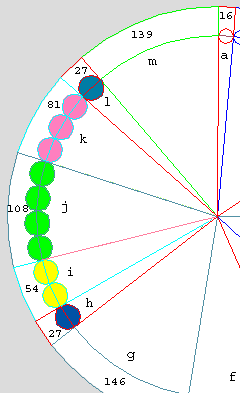the Rate of Precession The below link cites up to date (in 2006) information on precession. The heavenly mill precesses:                                .0139697 of one degree per year.  http://scienceworld.wolfram.com/physics/PrecessionoftheEquinoxes.html   A web search reveals utter confusion out there over the exact rate of precession, and the length of the Great Year (one precessional circle). Because the rate varies slightly during the course of precession, many writers out there seem convinced that it is impossible to put a value on the duration of one cycle other than an approximate one, such as 25,770 years +/- 100 years. Let Ancients be the judge! Being precession oriented, does the Frame have a pointer to the modern value given above?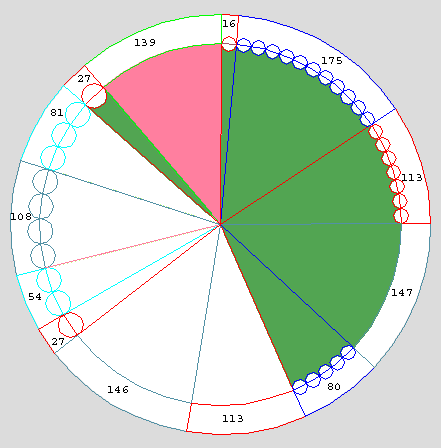There is a 139 segment on the Frame. It is part of the section of seven segments, whose total is 697 (see diagram above).  The Frame confirms the value for the rate of precession as given by the Astronomical Almanac in 2006. 360 degrees divided by .0139697 equals 25,770.0594.. years 146 x 175 got us to 25,776 with addition of the segments to their right.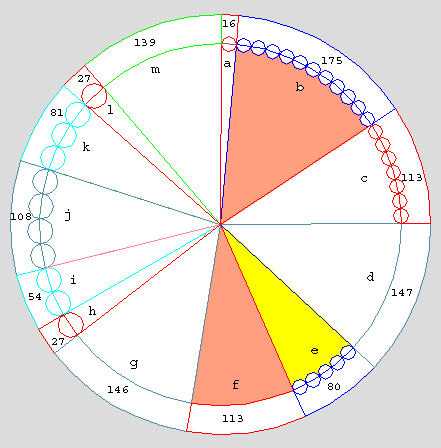Across the Strong Connection, ((175 + 113) : 80) : 139697  = 2.57700594... Those are the digits of the duration of one precessional cycle! Moreover, once we have the rate of precession, we don't have far to look for the 360 value to divide it into - we're looking at it! We're looking at the 360 degree circuit of the Frame.. 360 : .139697 = 2,577.00594...  or one-tenth of the precessional cycle Considering that all these values work with the clearly stated subject of equinoctial precession in the Frame, we have to respect these values once they show up. In effect, the Agency makes an important contribution to our knowledge. It has me convinced that the .0139697 value is the correct one. The fact carries an important implication: The average rate and duration of precession didn't change in 14,000 years, at least not enough to be noticed! The Frame is a magic circle: It spells deliberate order in almost every section of segments we choose to look at. We see layers and layers of meaning. All of it consistently refers to Pi, Phi, and the precession of equinoxes.  It justifies the work of professors Giorgio de Santillana, and Hertha von Dechend - Hamlet's Mill: An Essay Investigating the Origins of Human Knowledge And Its Transmission Through Myth - on precessional myths as indication of advanced prehistoric civilisation. http://www.bibliotecapleyades.net/hamlets_mill/hamletmill.htm Does theoretical thought coming to us in coded form from the depth of  ages warrant more research? It doesn't, according to the sum of all readers of this work, many of whom must be smart and educated. Whatever there is, must be all coincidence, which I had fished out of the stormy lake of chaos. Frankly, I'm insulted by that cliche. Speaking of coincidence, isn't it true that coincidence only goes so far? While any of the simple relationships on the Frame could occur randomly, we must not fail to see the system because of all the coincidences.  In fact, I find great comfort in that the Frame throws a gauntlet to critics, which they don't dare to pick up, because, if the Frame really were all coincidence, it should then be subject to improvement.            If the Frame's functions cannot be improved by rearranging, and replacing some, or even all of its thirteen numbers, well, then the Frame is the best solution in its category. This is a Game No Critic Dares to Play, worried about being dumber than alleged 'blind chance'. Such an observation must be particularly disconcerting to a professor of mathematics. Then perhaps this isn't an open-and-shut case of one lunatic against the world. This is prehistoric science versus what's bad with today's science, the willingness to cover up, or ignore unwanted anomalous evidence. Here, you have direct proof. It should be factored into the general discussion on the topic of advanced prehistoric science. Why would an agency eons ago bother creating these game-like time-capsules? Why play this multi-faceted game with us, if not for a very good, and perhaps vital reason? For worse, I remain the only player on this side. The burden of responsibility for the timely delivery of urgent prehistoric mail to humanity, from an unknown but powerful prehistoric agency, seems to rest on my narrow shoulders. Therefore, it's distinctly possible that I'll fail to deliver. For that I apologize,   ©  Jiri Mruzek mynameatyahoodotcom

Notes

 Game 8   The Frame & Cyclical Fractions & the Wheel of 113

355 / 113 = 3.141592..

I always marvel at how I had learned this approximation not from school, nor literature, but on my own, from Palaeolithic art. The 'cavemen', my Stone Age teachers also indicate knowing that the sixth Pi decimal in this particular formula is the last correct decimal.

The division by the prime number 113 yields a cyclical fraction, a repeating block of 112 digits. Every whole number from 1 to 112 appears once in this cycle, as the remainder (modulus).

The fractional cycle of 113 can be shown as a pie-chart - the "Wheel of 113".
We can look up all the segment values of the Frame on this chart by their remainder with 113.
Those seem to have no particular order on the chart, except that the sum of any two remainders diagonally opposed on the chart is always 113.

Numbers greater than 113, such as 139,  340, 887, 1000, or 1226, can be shown in successive layers around the wheel. For instance, we see that 1226, 1000, and 887 all leave the same remainder of 96, when divided by 113.
Because the sum of 146 & 80 is 113 x 2, the two are diametrically opposed across the chart.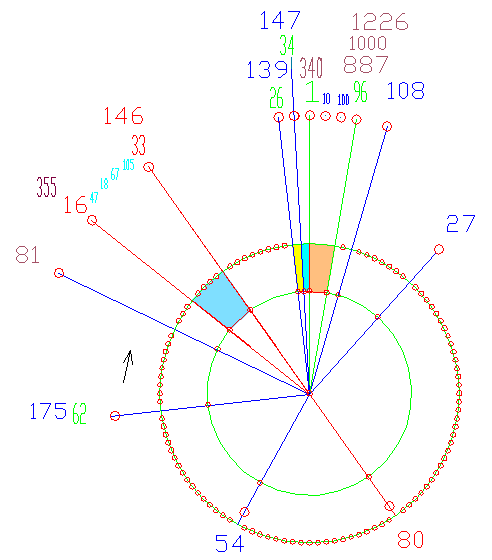Marking the start and the end of the cycle on the chart

Five of the ten Frame segment values
occupy a significant position on the Wheel of 113.
The start and the end of the cycle, is where we see an entire group of values:
The key first remainder in the cycle after the mandatory 1 & 10 & 100 & 1000 start  is 96. The total length of the Frame (1226) leaves this remainder.
Two Frame segment values (139 & 147) mark the last two steps of the cycle by their remainders. This dense grouping indicates the carrying out of all the 112 steps in the cycle.

The Pi-decimals

Two segments -  80 and 146 form a straight line across the pie-chart of 113, because they add up to twice 113.   We remember that in the image we also had a line subtending the section of 339, the Tri-balance, which is made of 80 and 146 sandwiching 113. That line was an obvious inner image divide..

The
sequence of six Pi digits on our pie-chart begins with 16, then 146 marks the sixth, and the last digit. So, 16, and 146 are markers for this sequence, and so is 80.
*
One marker for the start, and two markers for the end of the PI sequence on the pie-chart of 113 indicate that the Ancients knew not only where this PI approximation begins, but also where it ends.  To know that 355 / 113 gives Pi correct to the sixth decimal is to know that the seventh  decimal is wrong - and, how could one do that without knowing the correct seventh decimal of Pi?  As confirmation, we had Pi quoted, to eighteen decimals.

In addition to Pi and Phi, and the precessional (Osiris) numbers, we now have repeating fraction cycles, or blocks on the Frame's list of subjects.

Other interesting repeating blocks of  Frame values

80 / 81 = 0.  9  8  7  6  5  4  3  2   (repeating block)

1 / 81 = . 0  1  2   3  4   5  6  7  8 (repeating block)

80 & 81 are the lowest two whole numbers giving a ratio,  where decimals form a series of at least eight elements, descending by the regular interval of one.

1 & 81 are the lowest two whole numbers giving a ratio, where decimals form a series of at least nine elements, ascending by one.

80 and 81 total 161 -  the first three digits of Phi, or, the square root of  25,920 to within a hair 161 x 161 = 25,920 + 1.

80 x  81 = 6,480 = 1/4  of  25,920  (Years of the Zodiac)

*

Measuring and Remeasuring the Frame

I had neither a scanner, nor a computer back in 1985 to help me with graphics.

Once I got a high-res flatbed scanner, it was possible to import the image into CAD (computer assisted design). Then I had to regenerate its geometry:
I began with the Square, for I had long thought that it was very precise, and central. Then I added  the rest of the design over the Square, including the so called Cone (which sets the image's units of length, and is the progenitor of the Square).
My hand was utterly forced in this test, too, because there seems to be only one way of placing points of an exact square onto the engraved points. Finally, I surveyed the Frame in the terms of these newly derived units.

Success!
*
The Frame's total length of
1226 mms and eleven of its thirteen segments turned out the same, as before.. But one segment was two units (a millimeter in life-size) longer, and one that much shorter.
I suppose that a slight distortion had somehow happened at the printers, when printing the
blow-ups. The new, and for all I know, more accurate readings make much bettter sense, too. Basing on that, this version must be just like the ancients had intended it.

The Frame is simplicity itself, when compared to the rest of the image, and yet it is an ingenious mechanism. Anyone who learns the thirteen values of the Frame has in it a mnemonic device for a lot of little, but important bits of knowledge,  such as a full range of Pi and Phi approximations - or the key numbers for the Procession of Equinoxes.  It helps in memorizing things like how many nautical miles there are in the polar circumference of the Earth, or how many seconds there are in a 24 hour day, what the speed of light is in nautical miles per second, etc. In fact, familiarity with the Frame's ideas makes one aware of their strong presence in the modern world today, a valuable insight.

 Quote:  http://www-groups.dcs.st-and.ac.uk/~history/HistTopics/Pi_through_the_ages.html We know that π is not rational so there is no point from which the digits will repeat. However, if π is normal then the first million digits 314 159 26 535 8979... will occur from some point. Even if π is not normal this might hold! Does it? If so from what point? Note: Up to 200 million the longest to appear is 31415926 and this appears twice       .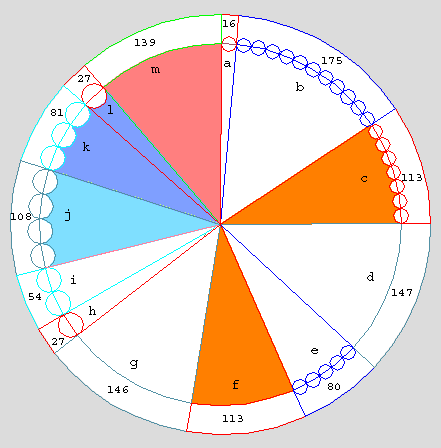Interesting tidbits  What is the square root of 216?    It is 14.6969,,,  Rounded to three digits,  it is 147.     Both 146, and 147  have some significance, when seen with 216,  What is the cubic root of 216?   6 x 6 x 6 = 216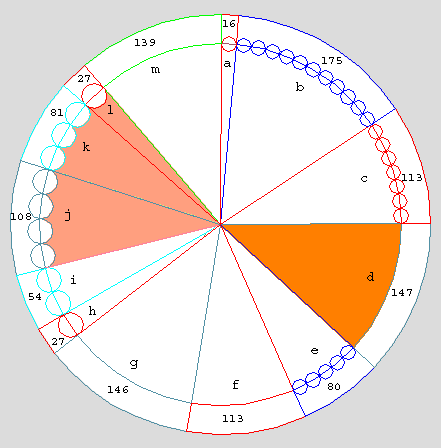The precessional cycle of Mars is 175,000  years.  Hmm, 175 is the Frame's highest value. Our sun sails around the galactic centre at 175 nautical miles per second. ----------------------------------------------------------- 175 is so far proving very versatile in operations yielding control values of Pi and Phi. It works with virtually everything around itself:  175 + 139         =   314    175 + 113         =   288  = 16 x 18   _______  1618,      Phi in four digits)  175                  =     35 x 5 or 355   _______  355 / 113 = 3.141592.. Pi to six decimals  175 - 113         =     62       0.62            Phi-minor rounded to two decimals    175 - 16           =   159       three digits in our readout of Pi decimals after 314     175 / 108         = 1.620..    Phi rounded to two decimals,     (175 / 108)  x 16 =  2,592   one-tenth of the classic precessional cycle We also see 175 heavily involved in all the  precessional modules, except one. The number 175 does everything.

 Top Menu Frame's Geometry - a) The HexMachine The Agency claims to have designed the layout of Giza's great pyramids An exact reconstruction of Giza's pyramidal layout as surveyed by W.F. Petrie Nasca Monkey External link - interesting - Pi man G H Bailey ©  Jiri Mruzek myname at yahoo dotcom                                                                                                  Vancouver, BC, Canada